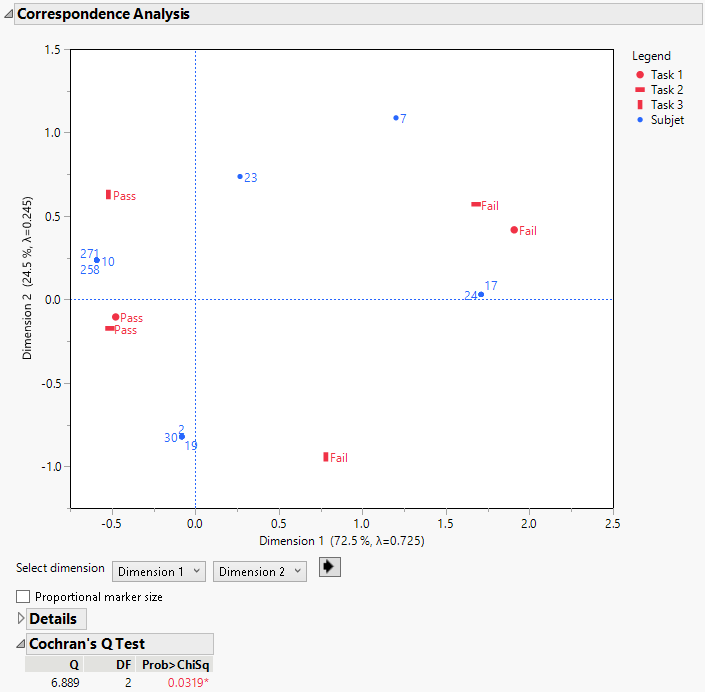Publication date: 08/13/2020

## Example of Cochran’s Q Test

This example uses a simulated data table to evaluate the ease of completion of three tasks for 30 subjects. Task 1 was simulated to have an 80% completion rate. Tasks 2 and 3 were simulated to have the same result as Task 1 for 95% and 80% of subjects respectively.

1. Select Help > Sample Data Library and open Cohcrans Q.jmp.

2. Select Analyze > Multivariate Methods > Multiple Correspondence Analysis.

4. Select Subject and click X, Factor.

5. Click OK.

6. Click the Multiple Correspondence Analysis red triangle menu and select Correspondence Analysis > Cochran’s Q Test.

Figure 7.8 Cochran’s Q TestThe correspondence plot shows the similarity between Task 1 and Task 2 as the Pass and Fail outcomes cluster together. However, Task 3 is farther away from Tasks 1 and 2. Cochran’s Q test statistic of 6.889 has an associated p-value of 0.0319. This p-value supports the rejection of the hypothesis that the pass rates are the same across all tasks at a 0.05 significance level.